NEET and AIPMT NEET Physics Kinetic Theory of Gases MCQ Questions Solved

An isolated system

(A) is a specified region where transfers of energy and mass takes place

(B) is a region of constant mass and only energy is allowed to close the boundaries

(C) is one in which mass within the system is not necessarily constant.

(D) cannot transfer either energy of mass to or from the surroundings.

(D) An isolated system is one which is separated so that mass and energy of the system cannot be transferred to or from the surroundings.

Difficulty Level:

• 10%
• 19%
• 6%
• 67%
PMT - 1997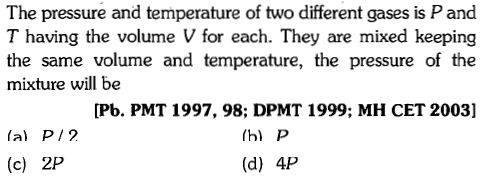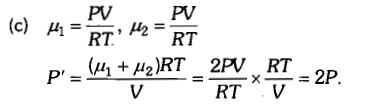Difficulty Level:

• 19%
• 45%
• 32%
• 7%
PMT - 2000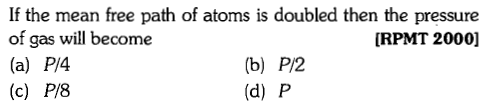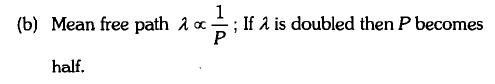Difficulty Level:

• 19%
• 51%
• 15%
• 18%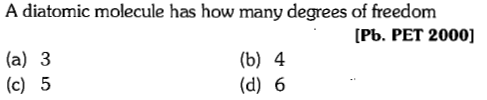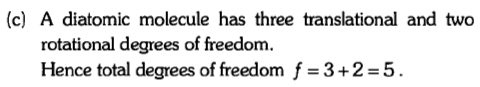Difficulty Level:

• 9%
• 14%
• 72%
• 6%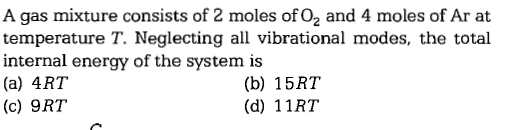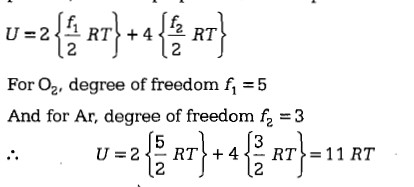Difficulty Level:

• 13%
• 18%
• 22%
• 49%

Which of the following relations is correct for root mean square speed of a gas at temperature T?

(A)     (B)

(C)     (D)

Where k= Bolzmann constant,

m= mass of a molecule.

(A)

Difficulty Level:

• 78%
• 15%
• 6%
• 4%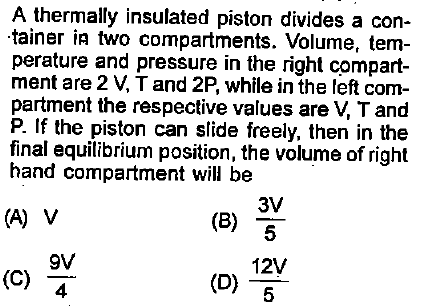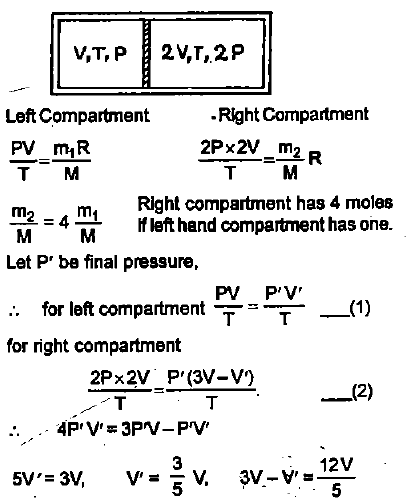Difficulty Level:

• 23%
• 35%
• 28%
• 17%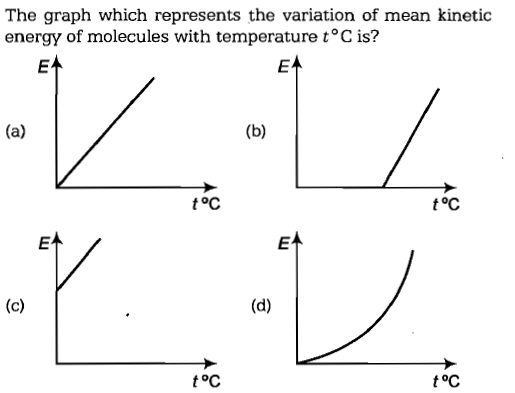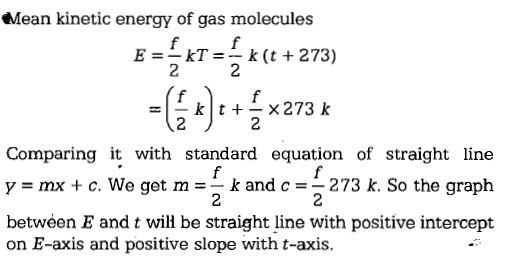Difficulty Level:

• 42%
• 15%
• 26%
• 19%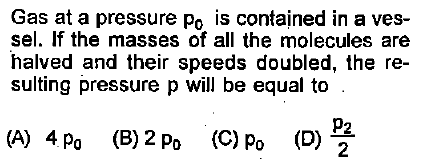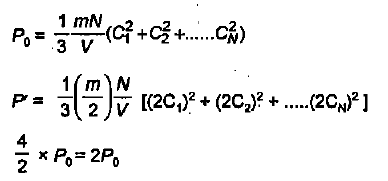Difficulty Level:

• 10%
• 56%
• 21%
• 16%

${V}_{rms},{V}_{av},{V}_{mp}$ are rrot mean square, averageand most probable speeds of molecules of a gas obeying Maxwellian velocity distribution. Which of the following statements is correct:

1. ${V}_{rms}<{V}_{av}<{V}_{mp}$

2. ${V}_{rms}>{V}_{av}>{V}_{mp}$

3.

4. ${V}_{avg}>{V}_{rms}<{V}_{mp}$

B option is Correct

Difficulty Level:

• 14%
• 46%
• 35%
• 7%